1620253250606370809018025086×86×5075×75×8075×75×40100×10065×6575×75×6065×95×5575×75×50110×110×60Φ65×16Φ65×20Φ65×2575×6050.8×106×6675×5075×7077×77×3877×77×5086×86×4065×4065×40×4065×65×2075×75×7077×77×7086×8686×86×6086×86×70164×77×38164×77×50200×200×10050.8×86×6686×86×8086×5086×86×4686×86×90100×100×80150×150×100150×150×70164×78×50172×86×50255×77×50300×200×10050.8×64×10350.8×64×10450.8×64×10750×1.260×1.265×40×1665×40×2065×40×2565×65×1665×65×2570×7075×75×7575×8077×77×4377×77×4877×77×6080×8086×6086×7086×8086×86×10086×86×54100×100×100105×105×50105×60×50106x61x50106×60×50107×61×50108×108×50118×118120×120×50123×77×35125×125×100125×125×60125×125×75125×175×100125×175×75138×65×50140×170×95141×80×50142×78×50143x61x50143×60×45143×60×50145×61×50146×146146×146×100146×146×50146×146×60146×146×70146×146×80146×146×90146×86×100146×86×50146×86×60146×86×70146×86×80146×86×90147×86×35150×150×150150×200×100150×200×130150×250×100150×250×130174×86×34175×175×110175×250×125178×65×50183×60×50184x61x50187×61×5018×24×1018×24×2618×24×2818×24×3018×24×3218×24×3318×24×3518×24×5018×24×52190×125×60200×100×80200×150×120200×150×150200×200×130200×200×150200×200×90200×260×50200×88×90242×190×108245×60×85250×150×120250×78×50253×78×50255×75×50258×86×50260×88×90270×190×108280×190×130280×260×50292×130×95318×255×108330×220×100330×430×100350×260×50373×255×10840×40422×338×110422×412×11450.8×64×8450.8×64×8550.8×64×8650×5050×64.5×10450×64×10350×64×10750×64×8450×64×8550×64×8650×65×5560×100×10060×108×5060×4560×6065×65×6565×70×6575×75×10075×75×34-D65/4075×75×35-D50/D6375×75×3875×75×8675×75×9075×9077x7777×7777×77×2277×77×3977×77×5477×77×5677×77×7880×110×4080×110×7080×110×8580×130×7080×130×8580×160×5580×160×9080×180×7080×180×8580×250×7080×250×8580×80×4580×80×4680×80×5081×81×5082×82×45860×860×60086x8686×86×3486×86×3586×86×4286×86×438×6×508×6×608×6×8090×9092×92×4597×65×50D16D20Φ33Φ40

2020201920182017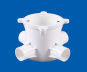13%
2020/11/26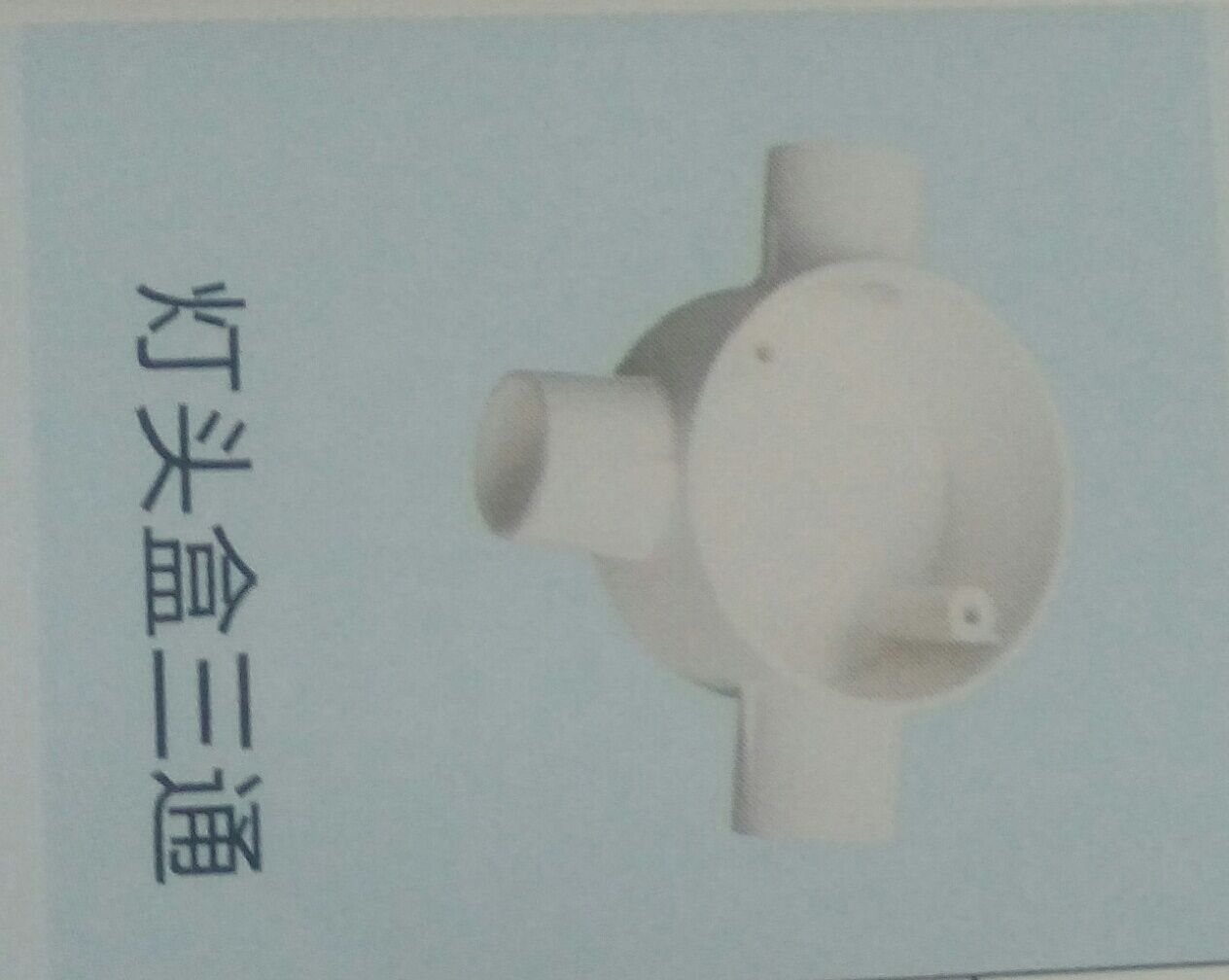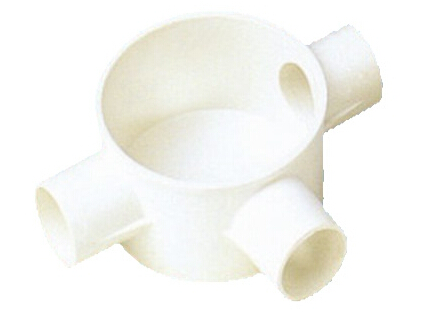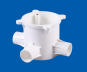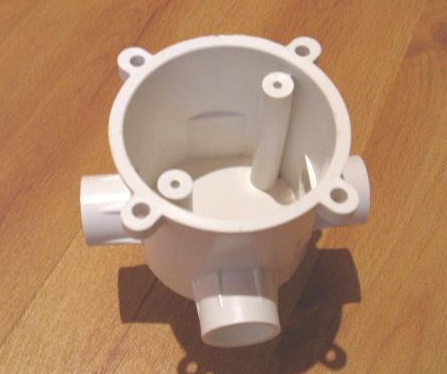13%
2020/11/26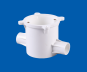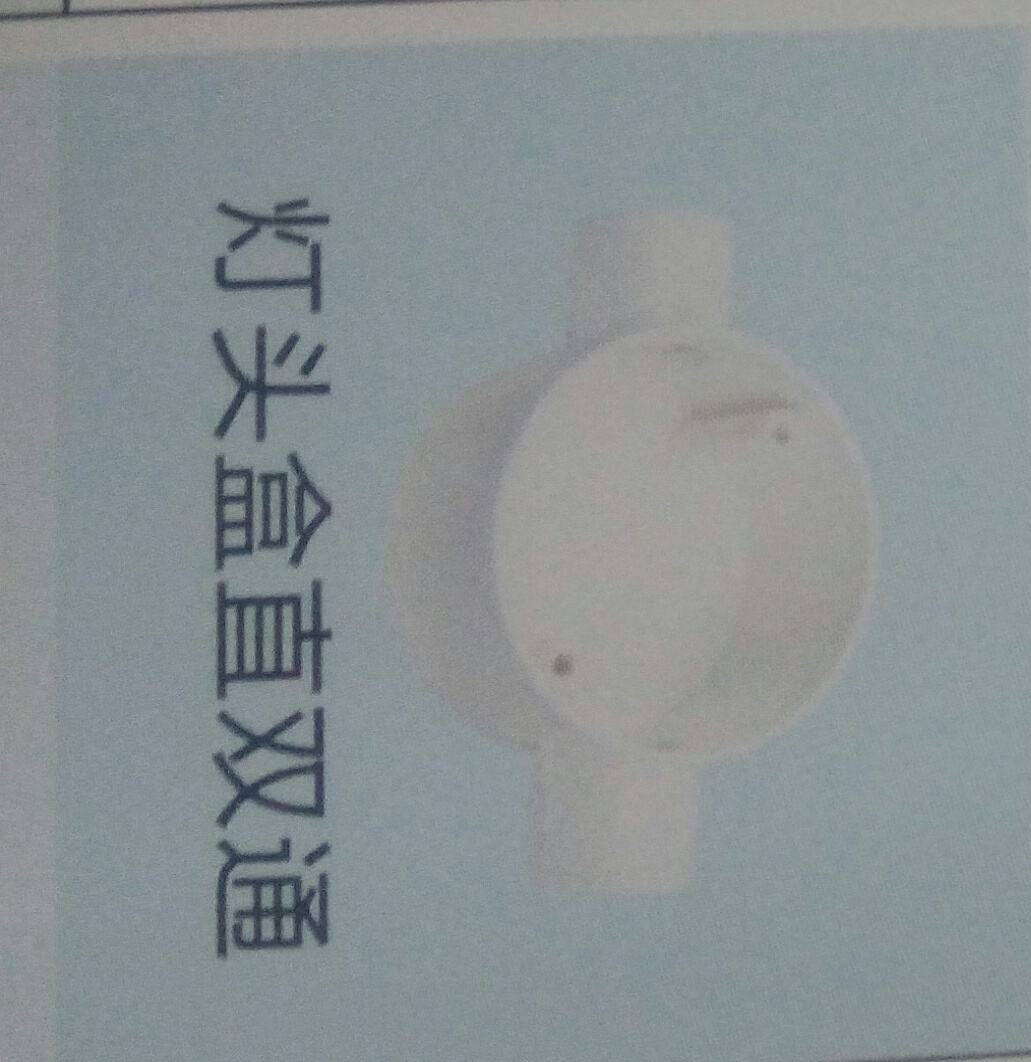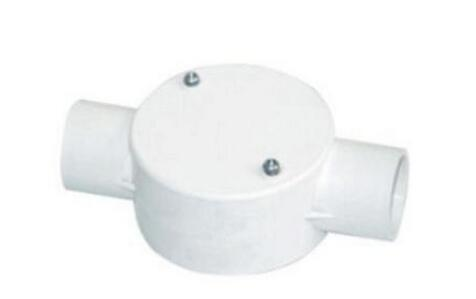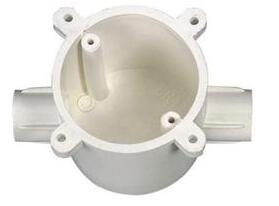13%
2020/11/26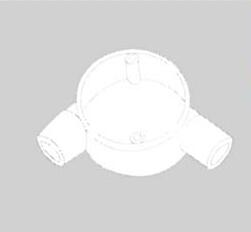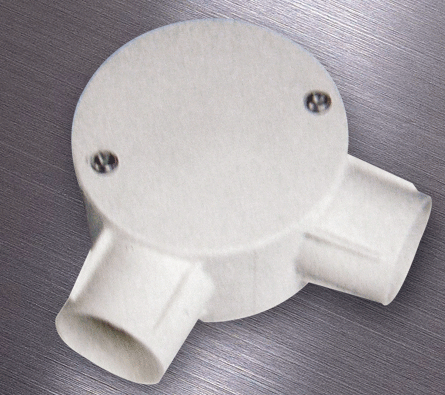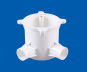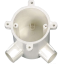13%
2020/11/26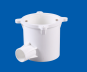13%
2020/11/26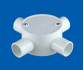13%
2020/11/26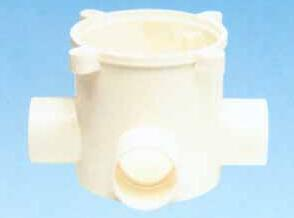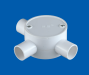13%
2020/11/26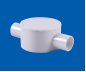13%
2020/11/26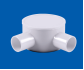13%
2020/11/26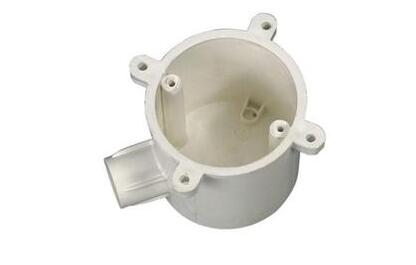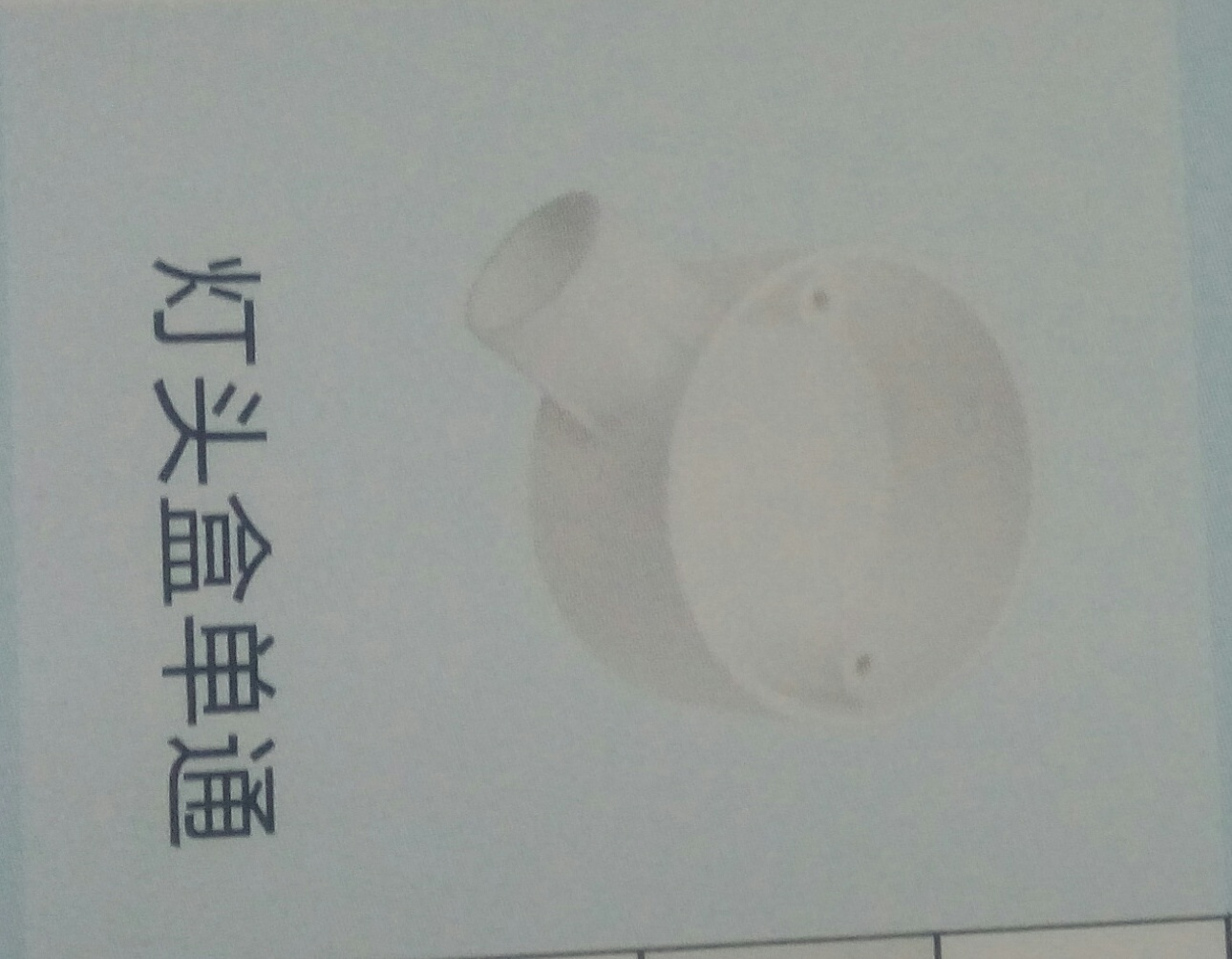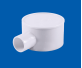13%
2020/11/26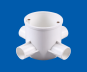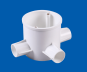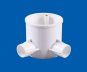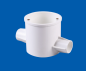13%
2020/11/26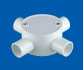13%
2020/11/26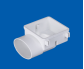13%
2020/11/26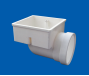13%
2020/11/26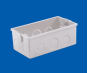13%
2020/11/26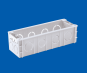13%
2020/11/26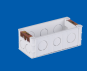13%
2020/11/26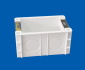13%
2020/11/26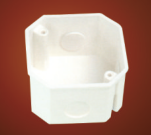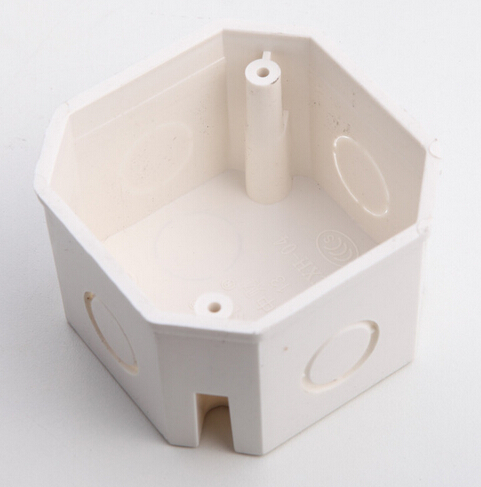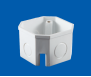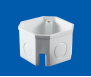13%
2020/11/2613%
2020/11/2613%
2020/11/2613%
2020/11/26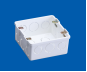13%
2020/11/26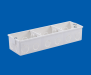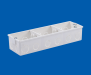13%
2020/11/26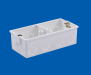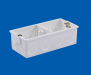13%
2020/11/26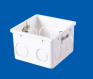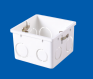13%
2020/11/26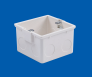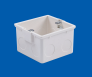13%
2020/11/26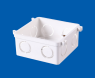13%
2020/11/26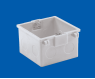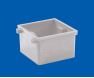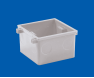13%
2020/11/26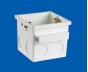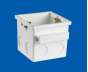13%
2020/11/26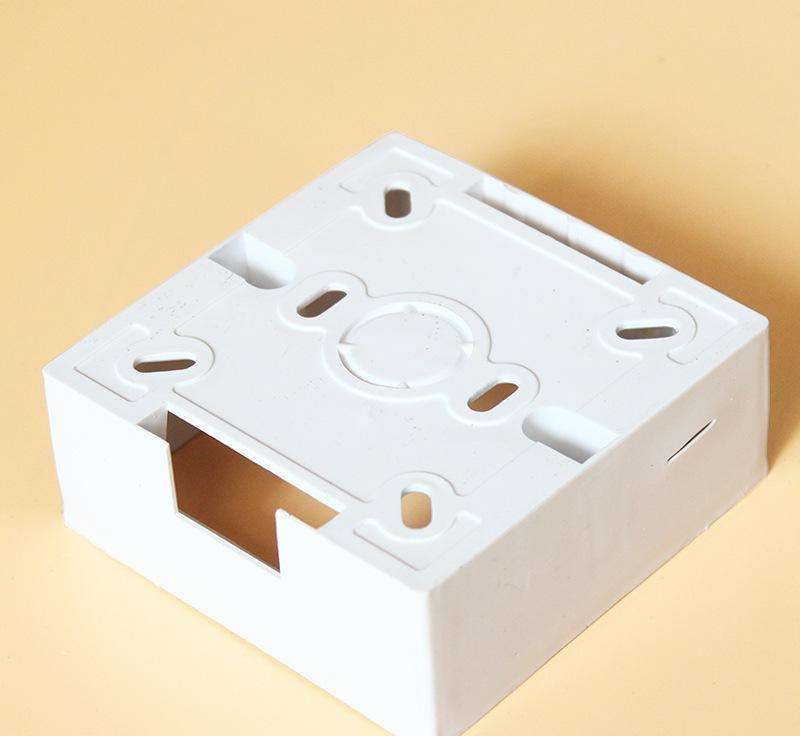13%
2020/11/2613%
2020/11/26

PVC-U三联线盒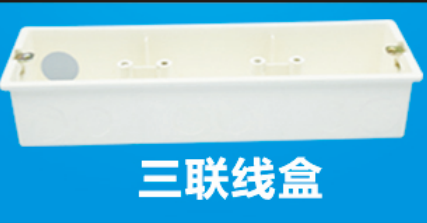13%
2020/11/26

PVC-U穿筋线盒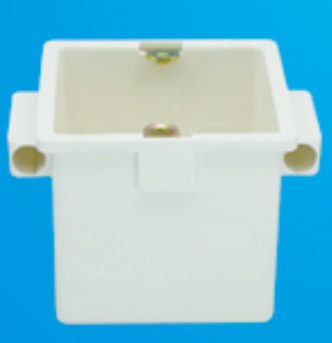13%
2020/11/26

PVC-U八角线盒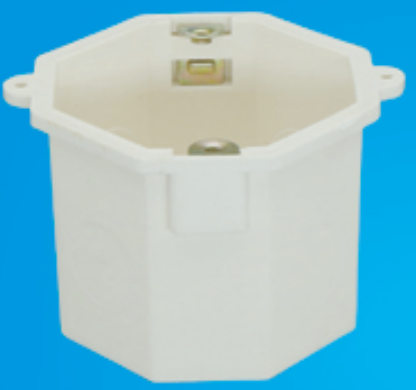13%
2020/11/26

PVC-U线盒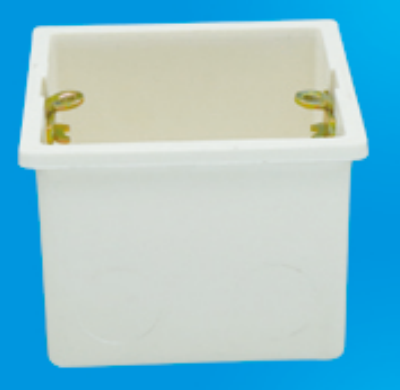13%
2020/11/26

PVC-U自攻丝线盒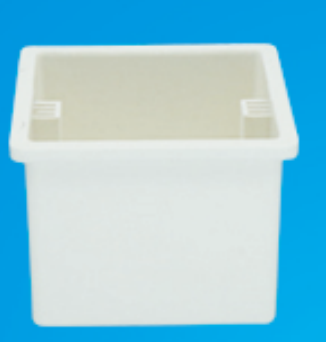13%
2020/11/26

PVC-U自攻丝线盒13%
2020/11/26

PVC-U穿筋线盒13%
2020/11/26

PVC-U拼装线盒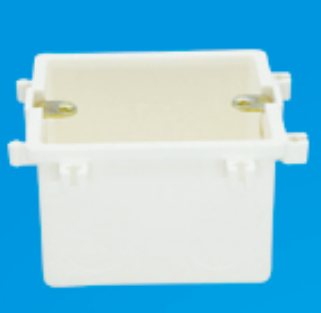13%
2020/11/26

• 1
• 2
• 3
• 4
• 5
• 6
• 100订阅中心广联达大数据